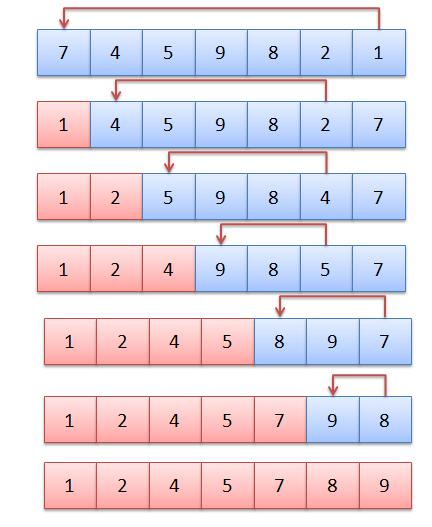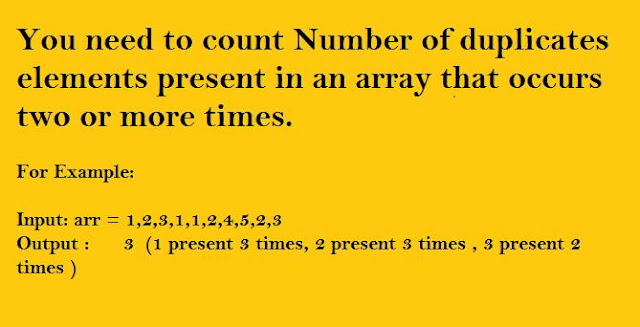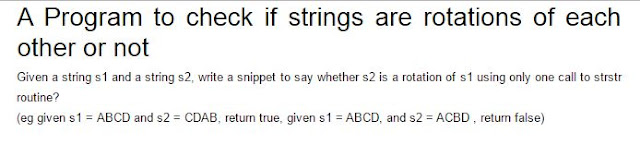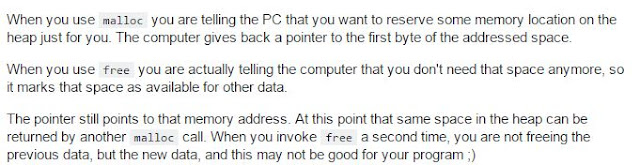# Selection Sort

Selection Sort

Selection sort is a simple sorting algorithm. This sorting algorithm is an in-place comparison-based algorithm in which the list is divided into two parts, the sorted part at the left end and the unsorted part at the right end. Initially, the sorted part is empty and the unsorted part is the entire list.Selection Sort

The smallest element is selected from the unsorted array and swapped with the leftmost element, and that element becomes a part of the sorted array. This process continues moving unsorted array boundary by one element to the right.

This algorithm is not suitable for large data sets as its average and worst case complexities are of Ο(n2), where n is the number of items.

How Selection Sort Works, refer link for more details

#include<iostream>
using namespace std;

class Selection
{
public:
void Selection_Sort(int a[],int n);
void Display_array(int a[], int n);
};

void Selection::Selection_Sort(int a[], int n)
{
int Amin=0;
for(int i=0;i<n-1;i++)
{
Amin=i;
for(int j=i+1;j<n;j++)
{
if(a[j]<a[Amin])
Amin=j;
}
int temp = a[i];
a[i]= a[Amin];
a[Amin]= temp;

}
}
void Selection::Display_array(int a[], int n)
{
cout<<"\n\n Sorted elements are :-"<<endl;
for(int i=0;i<n;i++)
{
cout<<a[i]<<" ";
}
cout<<"\n\n";
}
int main()
{
int a,n;
cout<<"\n\nenter no of elements:- ";
cin>>n;

cout<<"\nenter array elements:-"<<endl;
for(int i=0;i<n;i++)
{
cin>>a[i];
cout<<endl;
}
Selection obj;
obj.Selection_Sort(a,n);
obj.Display_array(a,n);

}

# Count Number Of Duplicates Elements Present In Array

Count Number Of Duplicates Elements Present In Array

You need to count Number of duplicates elements present in an array that occurs two or more times.

For Example:

Input: arr = 1,2,3,1,1,2,4,5,2,3
Output :      3  (1 present 3 times, 2 present 3 times , 3 present 2 times )Count-Duplicates-In-Array

#include<iostream>
#include<stdio.h>
using namespace std;
int main()
{
int arr[] = { 1,2,3,1,1,4,5,6,4,4,5,2,2,12};
int size1 = sizeof(arr)/sizeof(arr);
int count1 = 0,flag=0, temp = 0;;
int n = 0;

/*first sort array elements so that all duplicates will be next to each other*/

for (int i = 0; i<size1; i++)
{
for (int j = 0; j<size1 - i - 1; j++)
{
if (arr[j] > arr[j + 1])
{
temp = arr[j];
arr[j] = arr[j + 1];
arr[j + 1] = temp;
}
}
}

/*Print sorted array*/

for (int i = 0; i<size1; i++)
cout << arr[i] << "  ";
int i = 0;
while(i<size1)
{
flag = 0;
for (int j = i + 1; j<=size1; j++)
{
if (arr[i] == arr[j])
{
n++;
flag++;
if (flag ==1)
count1++;
continue;
/*if elements present the continue to next iteration inside nested loop*/
}
else
{
n++;
break;
}
}
i = n;
}
cout << "\n\n count value:-  " << count1;
getchar();

}

# Delete All Node From A Link List Greater Than A Given Value(X)

Delete All Node From Link List Greater Than A Given ValueLinked-List

Program:

{

node *prev,*temp,*temp1;
{
printf("\n\nlist is empty");
}
else if(ptr->data >x)
{
temp = ptr;
ptr=ptr->next;
free(temp);
}
else
{
temp = ptr;
prev = ptr;
printf("\n data :  %d",temp->data);
while(temp!=NULL)
{

if (temp->data >x)
{
temp1 = temp;
temp=temp->next;
prev->next = temp;
free(temp1);
temp =prev;

}
else
{
prev=temp;
temp=temp->next;
}
}

}
}

# A Program to check if strings are rotations of each other or not

A Program to check if strings are rotations of each other or notString-Rotations #include #include #include using namespace std; void CompareString(string, string, int); int ComputeLength(string str); int main() { string str = ""; string str1 = ""; int len = 0, len1 = 0; cout << "\nenter string "; cin >> str; cout << "\nenter string 2 to compare:-  "; cin >> str1; len = ComputeStringLength(str); len1 = ComputeStringLength(str1); if (len == len1) CompareString(str, str1, len); else cout << "rotation not possible"; getchar(); return 0; } int ComputeStringLength(string str) { int len = 0; for (int i = 0; str[i] != '\0'; i++) { len++; } return len; } void  CompareString(string str, string str1, int n) { int index = 0, flag = 0, curr_index = 0, count1 = 0, flagj = 0; for (int i = 0; i=0) { int k = 0; for (int i = n - 1; i>n - curr_index - 1; i--) { if (str[i] == str1[k]) { temp++; k++; } } } if (temp == n) { cout << "\n\nstring is same after rotation"; } else { cout << "\n\nstring is not same after rotation"; } } else { cout << "\n\nstring is same after rotation"; } } Other Blogs: Follow here

# Interview Question #1

What happens if you Call a free on a pointer twice?

Deallocating a memory area with free does not make the contents of the pointer NULL.

Suppose that you have int *a = malloc (sizeof (int)) and a has 0xdeadbeef and you execute free (a) then after execution a still contains 0xdeadbeef but after the free call this memory address is no more reserved for you.

Something like you have rented a flat with malloc used for some time, returned the flat by free then you might have a duplicate key for the flat, but it is not reserved for you.

Doing a free on an already freed memory will result in double free memory corruption.Callling-free-twice-on-pointer

Other Articles:

Article Sources For Learning

# Calculate Sum Of All Numbers Present In String

Calculate sum of all numbers present in a stringsum-of-all-digits-present-in-a-string

#include <iostream>
#include<stdio.h>
#include<string.h>
using namespace std;

int SumOfDigits(string str, int n);
int SumOfoverallNumberPresent_In_Sequence(string str, int n);

int main()
{
char str;

gets(str);
int length = strlen(str);
cout<<"\n\nsum of all digits present in string is :-  "<<SumOfDigits(str, length)<<endl;
cout<<"\n\nSum of different numbers present in string:-  "<<SumOfoverallNumberPresent_In_Sequence(str, length)<<endl;
return 0;
}

int SumOfDigits(string str, int n)
{
int i,sum=0,num=0;
for(i = 0;i<=n;i++)
{
if(str[i] >=48 && str[i] <= 57)
{
num = str[i]-48;
sum = sum  + num;
}

}
return sum;
}

int SumOfoverallNumberPresent_In_Sequence(string str, int n)
{
int i,sum=0,digitSum=0,Flag=0,num=0;
for(i = 0;i<=n;i++)
{
if(str[i] >=48 && str[i] <= 57)
{
num = str[i]-48;
sum = sum*10  + num;
}
else
{
digitSum = digitSum+sum;
sum=0;
}

}
return digitSum;
}

Other Articles:

Article Sources For Learning

# Kth smallest element in a row-wise and column-wise sorted 2D array

Kth smallest element in a row-wise and column-wise sorted 2D arrayKth-smallest-element

#include<iostream>
#include<stdio.h>
#include<climits>
using namespace std;

typedef struct HeapNode
{
int value;
int row;
int col;
}node;

void minHeapify(node arr[], int n ,int heap_size);
void swap_elements(node *x, node *y);
void  HeapSort(node arr[], int n);
int FindKthElement(int arr,int n,int k);

void swap_elements(node *x, node *y)
{
node z = *x;
*x= *y;
*y=z;
}

void minHeapify(node arr[], int n ,int heap_size)
{
int left = 2*n+1;
int right = 2*n+2;
int small =n;
if(left<heap_size && arr[left].value < arr[n].value)
small =left;
if(right<heap_size && arr[right].value < arr[small].value)
small =right;
if(small !=n)
{
swap_elements(&arr[small], &arr[n]);
minHeapify(arr,small,  heap_size);
}

}

void  HeapSort(node arr[], int n)
{
int i= (n-1)/2;
while(i>=0)
{
minHeapify(arr, i, n);
i--;
}
}

int FindKthElement(int  arr,int n,int k)
{
int next_value =0;
if(k<=0 || k>n*n)
return INT_MAX;

node temp_Arr[n];
/*creating heap for first row elements*/

for (int i = 0; i < n; i++)
temp_Arr[i] = {arr[i], 0, i};
HeapSort(temp_Arr, n);

node Next_value_array;

for(int i=0;i<k;i++)
{
Next_value_array = temp_Arr;

if(Next_value_array.row<(n-1))
{
next_value = arr[Next_value_array.row + 1][Next_value_array.col];
}
else
{
next_value = INT_MAX;
}
//int next_value = (Next_value_array.row<(n-1)) ? arr[Next_value_array.row + 1][Next_value_array.col]:INT_MAX;
temp_Arr =  {next_value, Next_value_array.row+1, Next_value_array.col };
minHeapify(temp_Arr,0, n);
}
return Next_value_array.value;
}

int main()
{
int arr = { {10, 20, 30, 40},
{15, 25, 35, 45},
{25, 29, 37, 48},
{32, 33, 39, 50},
};
cout << "7th smallest element is " << FindKthElement(arr, 4, 7);
return 0;
}

Other Articles: